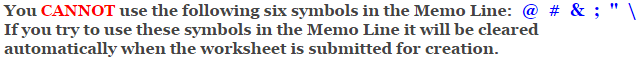# Calculus

## Differential Equation Worksheets

These Calculus Worksheets will produce problems that deal with finding the general solution to a differential equation.
The student will be given equations and will be asked to differentiate them.
You may select the number of problems, the types of equations to use, and the notation.

These Differential Equation Worksheets are a great resource for Differentiation Applications.

### Language for the Differential Equation Worksheets

 English German Albanian Spanish Swedish Italian French Turkish Polish Norwegian

### Memo Line for the Differential Equation Worksheets

You may enter a message or special instruction that will appear on the bottom left corner of the Differential Equation Worksheets.### Differential Equation Worksheets Answer Page

Now you are ready to create your Differential Equation Worksheets by pressing the Create Button.

 Recommended Videos

If You Experience Display Problems with Your Math Worksheet